# Deeplearning之CNN类型总结

## 经典网络、深度网络、目标识别网络

Posted by Yunlongs on September 21, 2019

# Deeplearning之CNN类型总结

## 一.经典网络

### 1. LeNet-5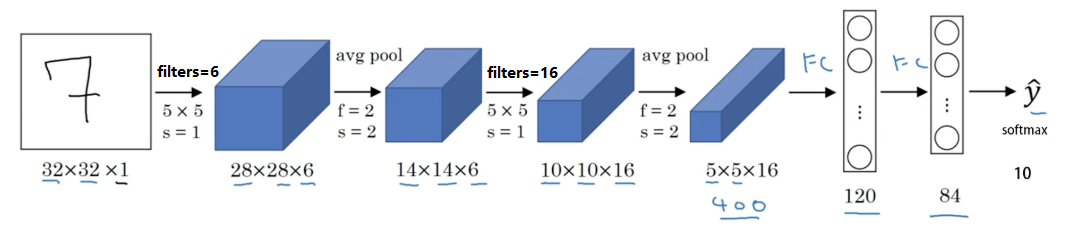• 针对灰度图片训练的，所以图片的大小只有32×32×1
• 包含大约6w个参数
• 现在一般使用 ReLU 作为激活函数，输出层一般选择 softmax。

### 2. AlexNet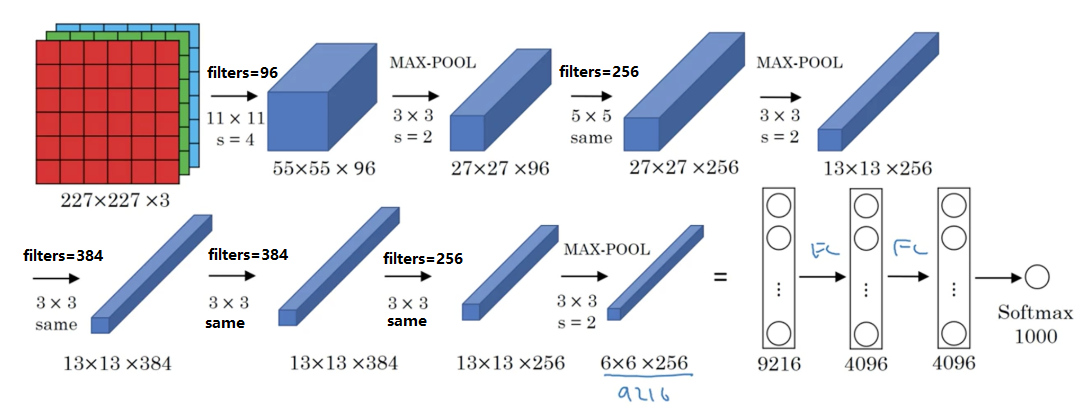• 约6000w个参数
• 激活函数为 RELU

### 3. VGG-16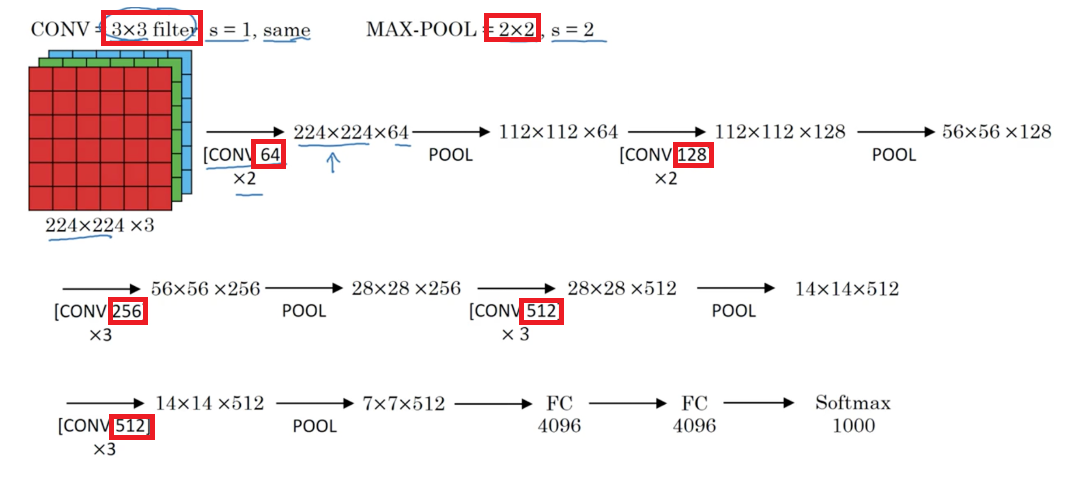• 简化了神经网络结构，1.38亿个参数
• 网络结构非常规整，卷积层大小都为$3 \times 3$，且逐步将通道数扩大一倍；池化层大小都为$2 \times 2$，用来逐步缩小图像尺寸一倍。
• VGG-16中的16代表，可以训练的层数为16层（卷积层+FC层+softmax层）
• 缺点是，需要训练的特征数量非常巨大

## 二.深度卷积网络

### 1. 残差网络（ResNet-50）

$a^{[l]}$与$a^{[l+2]}$之间隔层联系的表达式为： $a^{[l+2]}=g\left(z^{[l+2]}+a^{[l)}\right)$

ResNet-50的网络结构如图：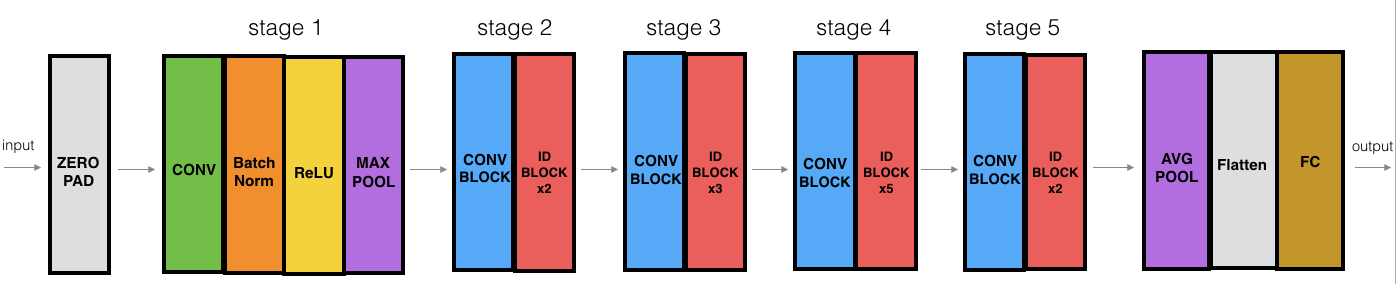• 阶段一
• 卷积层，大小为$7 \times 7$的64个filters，$strides = (2,2)$
• 最大池层，大小为$3 \times 3$,$strides = (2,2)$
• 阶段二
• 3层卷积层，大小为$3 \times 3$，每一层filters的数量分别为$[64,64,256]$
• 2个ID BLOCK，规模和前一卷积块一样
• 阶段三
• 三层卷积层，大小为$3 \times3$,$strides=(2,2)$,每一层的filters的数量分别为$[128,128,512]$
• 3个ID BLOCK，规模和前一卷积块一样
• 阶段四
• 三层卷积层，大小为$3 \times 3$,$strides=(2,2)$,每一层的filteres的数量分别为$[256,256,1024]$
• 5个ID BLOCK，规模和前一卷积块一样
• 阶段五
• 三层卷积层，大小为$3 \times 3$,$strides = (2,2)$,每一层的filters的数量分别为$[512,512,2048]$
• ## 2个ID BLOCK，规模和前一卷积块一样

1. 引入矩阵$W_s$与$a^{[l]}$相乘，使其维度与$a^{[l+2]}$一致
• 将$W_s$作为学习参数，通过模型训练得到
• 固定$W_s$的值，不够补0

### 2.谷歌 Inception

Inception单元如图：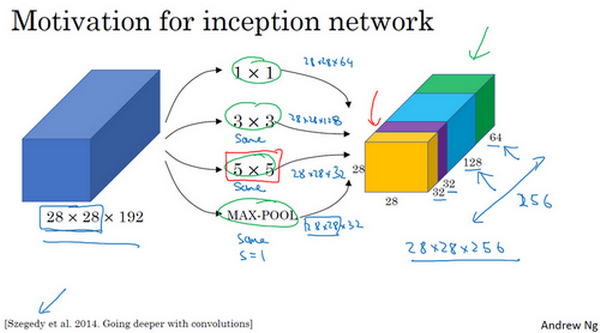• 不需要人工来确定卷积层中的 过滤器类型，和是否需要创建卷积层或者池化层。
• 所有的filters都需要进行same填充
• 计算量巨大，可以使用$1 \times 1$的卷积进行减少计算量

## 1. YoLo算法

• 并没有在3×3网格上跑9次算法，而是单次卷积实现，但在处理这3×3计算中很多计算步骤是共享的，所以这个算法效率很高
• 显式地输出边界框坐标，可以具有任意宽高比，并且能输出更精确的坐标，不会受到滑动窗口分类器的步长大小限制
• 因为是卷积实现，运行速度非常快，可以达到实时识别

### 2.R-CNN算法

R-CNN算法首先使用K-Means聚类算法，对图像进行聚类处理，在每个色块上进行处理，然后再分类。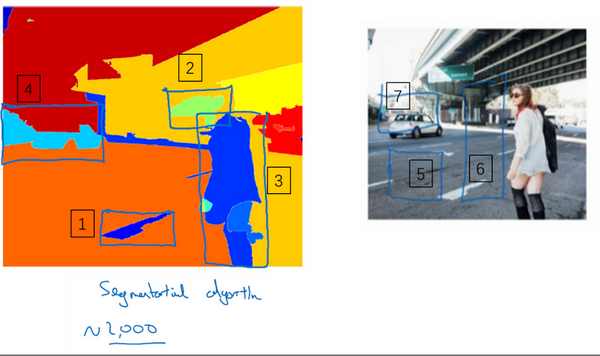• 算法运行速度很慢，每次分类前都需要对图像进行处理。

Fast R-CNN Fast R-CNN算法基本上是R-CNN算法，最初的算法是逐一对区域分类，快速R-CNN用的是滑动窗法的一个卷积实现，显著提升了R-CNN的速度，问题是得到候选区域的聚类步骤仍然非常缓慢

Faster R-CNN 更快的R-CNN算法（Faster R-CNN），使用的是卷积神经网络，而不是更传统的分割算法来获得候选区域色块，比Fast R-CNN算法快得多。 不过大多数更快R-CNN的算法实现还是比YOLO算法慢很多